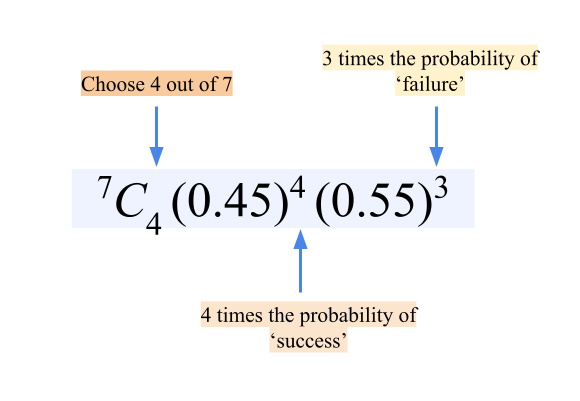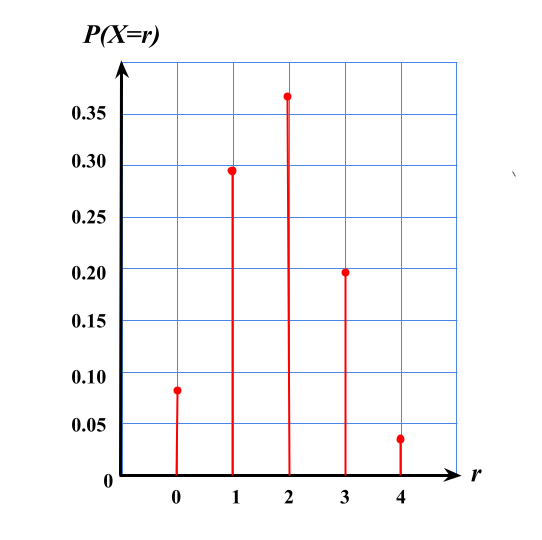Binomial Distribution

 5.2 Binomial Distribution

Bernoulli trial

 An experiment that produces only two possible outcomes.

Characteristics of Bernoulli Trials
 There are only two possible outcomes, namely 'success' and 'failure' The chances of ‘success’ are always the same in every trial If the probability of 'success' is given by $$p$$, then the probability of 'failure' is given by $$(1-p)$$ where $$0 \ \text{<} \ p \ \text{<} 1$$ The discrete random variable $$X = \lbrace 0, 1 \rbrace,$$ where $$0$$ represents 'failure' and $$1$$  represents 'success'

• A binomial random variable is the number of success $$r$$ from $$n$$ similar Bernoulli trials in a binomial experiments
• The probability distribution of a binomial random variable is known as a binomial distribution
• An experiment with $$n$$ equals to $$1$$ is a Bernoulli trial

 Example A shelf contains $$6$$ identical copies of chemistry reference books and $$4$$ identical copies of physics reference books. $$3$$ copies of the physics reference books are taken at random from the shelf one after another without replacement. State whether this probability distribution is a binomial distribution or not. Explain. Solution: $$P(\text{getting the 1st copy of physics reference book)}\\ = \dfrac{4}{10}= \dfrac{2}{5}$$ $$P(\text{getting the 2nd copy of physics reference book)}\\ = \dfrac{3}{9}= \dfrac{1}{3}$$ $$P(\text{getting the 3rd copy of physics reference book)}\\ = \dfrac{4}{8}= \dfrac{1}{4}$$ The probability of getting a copy of the physics reference book in each trial changes and each outcome depends on the previous outcome. Thus, it is not a binomial distribution.

• The binomial probability function for $$X$$ can be written as $$X \sim \text{B}(n,p)$$ given by the following formula:

 $$P(X=r) = \ ^{n}C_{r}p^rq^{(n-r)}, \ r=1, \ 2, \ 3, \ ..., \ n$$

Remark
 $$^{n}C_{r}$$ means that there are $$r$$ 'success' in $$n$$ trials

• The total probability for the random variable $$X$$ in general is

 $$\sum_{i=1}^{n}\ P(X = r_i) = 1$$

Example

The probability that it rains on a certain day is $$0.45$$.

By using the formula, find the probability that in a particular week, it rains.

 (a) exactly $$4$$ days, (b) at least $$2$$ days. Solution: Let $$X$$ represent the number of rainy days. Given $$n = 7, p = 0.45$$ and $$q = 0.55$$. (a) \begin{aligned} P(X=4) &= \ ^{7}C_{4}(0.45)^4(0.55)^3\\ &= 0.2388 \end{aligned} (b) \begin{aligned} P(X \geqslant 2) &= P(X=2) + P(X=3)+ P(X=4) \\ & \ \ \ \ +P(X=5)+P(X=6)+P(X=7)\\\\ &= 1-[P(X=0)+P(X=1)]\\\\ &= 1- [\ ^{7}C_{0}(0.45)^0(0.55)^7 + \ ^{7}C_{1}(0.45)^1(0.55)^6]\\\\ &= 1-0.0152-0.0872\\\\ &=0.8976 \end{aligned}• The probability of the random variable $$X$$ of a binomial distribution can be obtained from the table as well as from the probability distribution graph

• For any $$n$$ of a binomial distribution:

 When $$p=0.5$$, the graph is symmetrical. When $$p\text{ < }0.5$$, the graph is skewed to the left and is not symmetrical. When  $$p\text{ > }0.5$$, the graph is skewed to the right and is not symmetrical.

Example

Emma did a survey on the percentage of pupils in her school who use school buses to come to school.

It is found that $$45\%$$ of pupils from her school use school buses.

A sample of $$4$$ pupils is randomly selected from the school.

(a)

Construct a binomial probability distribution table for the number of pupils who use school bus.

(b)

Draw a graph for this distribution.

(c)

From the table or graph, find the probability that

 (i) more than $$3$$ pupils come to school by school buses, (ii) less than $$2$$ pupils use school buses.

Solution:

(a)

Let $$X$$ epresent the number of pupils who use school buses.

Then, $$X = \lbrace 0, 1, 2, 3, 4\rbrace$$.

Given $$n=4, \ p=0.45 \text{ and }q=0.55$$.

 $$X=r$$ $$P(X=r)$$ $$0$$ $$^{4}C_{0}(0.45)^0(0.55)^4 = 0.0915$$ $$1$$ $$^{4}C_{1}(0.45)^1(0.55)^3 = 0.2995$$ $$2$$ $$^{4}C_{2}(0.45)^2(0.55)^2 = 0.3675$$ $$3$$ $$^{4}C_{3}(0.45)^3(0.55)^1 = 0.2005$$ $$4$$ $$^{4}C_{4}(0.45)^4(0.55)^0 = 0.0410$$

(b)(c)
 (i) \begin{aligned} P(X\text{ > }3) &= P(X=4)\\ &=0.0410 \end{aligned} (ii) \begin{aligned} P(X\text{ < }2) &= P(X=0) + P(X=1)\\ &=0.0915+0.2995\\ &=0.3910 \end{aligned}

• If a discrete random variable $$X$$ has a binomial distribution, that is, $$X \sim\text{B}(n,p)$$, then the expected value or mean, $$\mu$$ can be defined as:

Expected Value or Mean, $$\mu$$
 The sum of the product of the value of $$X$$ with its respective probability divided by the total probability of the distribution.

 $$\mu = \dfrac{\sum_{i=0}^{n}\ r \ P(X = r)}{\sum_{i=0}^{n}\ P(X = r)}$$

• Since $$\sum_{i=0}^{n}\ P(X = r) = 1$$, then the mean, $$\mu$$:
 $$\mu =np$$

• Variance, $$\sigma^2$$:
 $$\sigma^2 =npq$$

• Standard deviation, $$\sigma$$ is a measure of deviation of a set of data from its mean value:
 $$\sigma=\sqrt{npq}$$

 Example A study shows that $$95\%$$ of Malaysians aged $$20$$ and above have a driving license. If $$160$$ people are randomly selected from this age group, estimate the number of Malaysians aged $$20$$ and above who have a driving license. Then, find the variance and the standard deviation of the distribution. Solution: Given $$p = 0.95, \ q = 0.05 \text{ and } n = 160.$$ \begin{aligned} \text{Mean, } \mu&=np\\ &=160\times 0.95\\ &= 152 \end{aligned} \begin{aligned} \text{Variance, } \sigma^2 &=npq\\ &=160\times 0.95 \times 0.05\\ &= 7.60 \end{aligned} \begin{aligned} \text{Standard deviation, } \sigma&=\sqrt{npq}\\ &=\sqrt{7.60}\\ &= 2.76 \end{aligned}